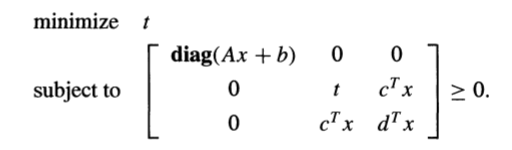# SDP complexity with vector variable

Hi!

I am trying to get the complexity of the SDP problem for my specific problem, but I’m facing some problems. Due to confidentiality, I can’t post my problem, but I’ll try my best to have an example that answers my questions.

I found in the literature that the complexity of the SDP problem for interior point per iteration is O(m * n^3 + m^2 * n^2 + m^3), where m is the number of constraints with (n x n) semidefinite matrix. It also implies that this (n x n) semidefinite matrix is the problem variable by saying there are n^2 variables.

This is where my problem starts because I don’t have a matrix variable, I have a 2x1 main variable. Furthermore, I have several auxiliary variables (vectors also) that other authors seem to neglect on the complexity, not sure if there’s a reason? I’m working in SDP mode, so my question is: is the problem transformed to have matrix variables at some point?

The main variable (of size 2x1) appears in one of the two SDP constraints I have, which is of size 3x3. Is it reasonable to say that n = r+1 where r = 2 is the number of variables?

Consider the following problem introduced by Boyd and Vandenberghe in https://web.stanford.edu/~boyd/papers/pdf/semidef_prog.pdf (page 3, from eqs 3 to 5).Neither variable is a matrix, there’s a vector variable x and a scalar variable t. Also, when I solved the problem with cvx, it says there are 10 variables and 3 equality constraints. It’s my first time dealing with SDP programming, so I’m finding this a bit mysterious. I have seen somewhere in this forum that the problem is indeed transformed in order to be solved, so the original 3 variables + 1 constraint become another thing. Is it possible to see such transformation? Or can someone point me to some reference that explains the transformations? And what would be the complexity in this case? Should I use the transformed problem (10 and 3) or the original problem (3 and 1)?

About my problem, if it matters: it starts as a problem with two uncertainties, both of them in the objective function. I added an auxiliary variable to pass those uncertainties to a constraint. Then I separate each uncertainty into two constraints (more auxiliary variables) and applied the S-procedure (even more auxiliary variables) to each so that they are now semidefinite constraints (LMI). For both LMI’s there are K constraints, where K is a fixed number. One LMI is 2x2 (uncertainty and auxiliary variables) and the other is 3x3 (main variable and auxiliary variables). Then I have constraints on auxiliary variables to be positive, a linear constraint on one of the auxiliary variables and a convex constraint for the main variable.
I thought that maybe I could use m = K (the rest is just scalar, can be removed from big-O, I think) and n = r+1, where r = 2 is the number of variables, but now I’m not even sure if I can use that formula above…

I’m aware that part of this may be a bit out of the scope of this forum, but I hope there’s someone out there who can help me with this.

Thanks and sorry for the long text!